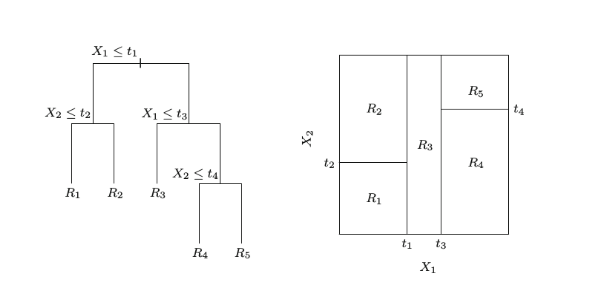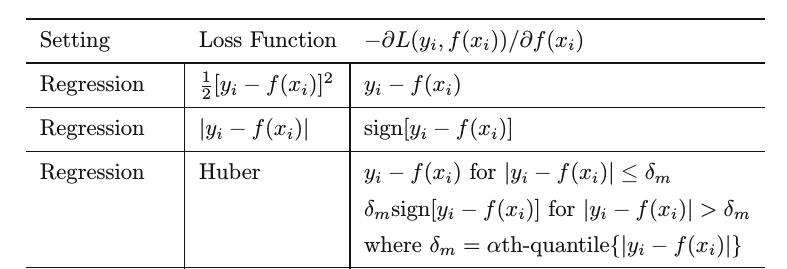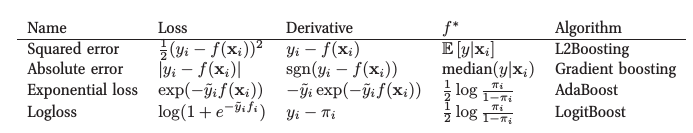#0
0
01. 云栖社区>
2. 博客>
3. 正文

massquantity 2018-06-13 17:34:00 浏览1468

## 函数空间的的梯度下降

$\theta = \theta - \alpha \cdot \frac{\partial}{\partial \theta}L(\theta) \tag{1.1}$

Gradient Boosting 采用和AdaBoost同样的加法模型，在第m次迭代中，前m-1个基学习器都是固定的，即$f_m(x) = f_{m-1}(x) + \rho_m h_m(x) \tag{1.2}$

$f_m(x) = f_{m-1}(x) - \rho_m \cdot \frac{\partial}{\partial f_{m-1}(x)}L(y,f_{m-1}(x)) \tag{1.3}$

1. 初始化： $$f_0(x) = \mathop{\arg\min}\limits_\gamma \sum\limits_{i=1}^N L(y_i, \gamma)$$

2. for m=1 to M:

(a) 计算负梯度： $$\tilde{y}_i = -\frac{\partial L(y_i,f_{m-1}(x_i))}{\partial f_{m-1}(x_i)}, \qquad i = 1,2 \cdots N$$

(b) 通过最小化平方误差，用基学习器$$h_m(x)$$拟合$$\tilde{y_i}$$$$w_m = \mathop{\arg\min}\limits_w \sum\limits_{i=1}^{N} \left[\tilde{y}_i - h_m(x_i\,;\,w) \right]^2$$

(c) 使用line search确定步长$$\rho_m$$，以使$$L$$最小，$$\rho_m = \mathop{\arg\min}\limits_{\rho} \sum\limits_{i=1}^{N} L(y_i,f_{m-1}(x_i) + \rho h_m(x_i\,;\,w_m))$$

(d) $$f_m(x) = f_{m-1}(x) + \rho_m h_m(x\,;\,w_m)$$

3. 输出$$f_M(x)$$

## 回归提升树$\left \{ R_{jm} \right\}_1^J = \mathop{\arg\min}\limits_{\left \{ R_{jm} \right\}_1^J}\sum\limits_{i=1}^N \left [\tilde{y}_i - h_m(x_i\,;\,\left \{R_{jm},b_{jm} \right\}_1^J) \right]^2$

$\gamma_{jm} = \mathop{\arg\min}\limits_\gamma \sum\limits_{x_i \in R_{jm}}L(y_i,f_{m-1}(x_i)+\gamma)$

## GBDT回归算法流程

1. 初始化： $$f_0(x) = \mathop{\arg\min}\limits_\gamma \sum\limits_{i=1}^N L(y_i, \gamma)$$

2. for m=1 to M:

(a) 计算负梯度： $$\tilde{y}_i = -\frac{\partial L(y_i,f_{m-1}(x_i))}{\partial f_{m-1}(x_i)}, \qquad i = 1,2 \cdots N$$

(b) $$\left \{ R_{jm} \right\}_1^J = \mathop{\arg\min}\limits_{\left \{ R_{jm} \right\}_1^J}\sum\limits_{i=1}^N \left [\tilde{y}_i - h_m(x_i\,;\,\left \{R_{jm},b_{jm} \right\}_1^J) \right]^2$$

(c) $$\gamma_{jm} = \mathop{\arg\min}\limits_\gamma \sum\limits_{x_i \in R_{jm}}L(y_i,f_{m-1}(x_i)+\gamma)$$

(d) $$f_m(x) = f_{m-1}(x) + \sum\limits_{j=1}^J \gamma_{jm}I(x \in R_{jm})$$

3. 输出$$f_M(x)$$

## 回归问题损失函数的选择## 分类提升树## 正则化 (Regularization)

### 1、Shrinkage

$f_m(x) = f_{m-1}(x) + \rho_m h_m(x\,;\,w_m)$

$f_m(x) = f_{m-1}(x) + \nu \rho_m h_m(x\,;\,w_m)$

### 4、Subsampling

subsampling的另一个好处是因为只使用一部分样本进行训练，所以能显著降低计算开销。

## Reference:

1. Friedman, J. Greedy Function Approximation: A Gradient Boosting Machine
2. Friedman, J. Stochastic Gradient Boosting
3. Friedman, J., Hastie, T. and Tibshirani, R. The Elements of Staistical Learning

/

massquantity
+ 关注